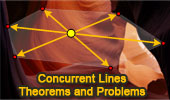# Geometry: Concurrent Lines, Theorems and Problems

## Concurrent Lines - Table of Content - Page 5Isogonic-Jacobi Theorem: Using TracenPoche Dynamic Geometry Software.
Concurrent lines.

Marion Walter's Theorem: Triangle and Hexagon areas: Using TracenPoche Dynamic Geometry Software. Concurrent Lines?

Nine-Point Center, Nine-Point Circle, Euler Line.
Interactive illustration.
Concurrent altitudes, median, perpendicular bisectors.

Seven Circles Theorem
Concurrent lines of tangency points.

Proposed Problem 79: Triangle. Similarity, Concurrent Altitudes, Orthocenter, Incircles, Inradii.

Triangle and Squares 22
Concurrent altitudes.

Proposed Problem 51: Fagnano's Problem
Inscribed Triangle with the Minimum Perimeter.
Concurrent altitudes.

Spieker Center
Concurrent lines.

Second Ajima-Malfatti Point
Interactive illustration. Concurrent lines.

First Ajima-Malfatti Point
Animated illustration. Concurrent lines.

Triangle and Squares 17
Concurrent lines.

Triangle and Squares 16
Concurrent lines.

Blanchet Theorem
Concurrent lines.

Proposed problem 21
Acute triangle, orthocenter, diameter, tangents. Concurrent altitudes.

Go to page: Previous | 1 | 2 | 3 | 4 | 5 | Next